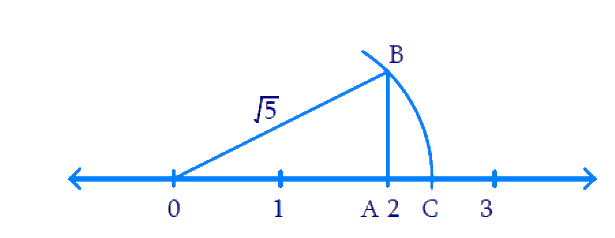# Exercise 1.2 Number Systems NCERT Solutions Class 9

## Chapter 1 Ex.1.2 Question 1

State whether the following statements are true or false. Justify your Answers.

### Solution

(i) Every irrational number is a real number.

Steps:

True, because the set of real numbers consists of rational numbers and irrational numbers.

(ii) Every point on the number line in of the form \begin{align}\,\sqrt m ,\end{align} where $$m$$ is a natural number.

Steps:

False, for example \begin{align}\sqrt {\frac{2}{3}} \end{align} is a real number on the number line but \begin{align}\frac{2}{3}\end{align} is not a natural number (Note that \begin{align}\frac{2}{3}\end{align} is a rational number).

(iii) Every real number is an irrational number.

Steps:

False. Note that the set of real numbers contains both rational and irrational numbers. As an example, \begin{align}\frac{1}{2}\end{align} is a rational number and hence it is real. But it is not an irrational number.

## Chapter 1 Ex.1.2 Question 2

Are the square roots of all positive integers irrational? If not, give an example of the square root of a number that is a rational number.

### Solution

Steps:

The square roots of positive integers are not irrationals.

Example: \begin{align}\,\sqrt 4 = 2\end{align} and $$2$$ in a rational number \begin{align} \left(\because 2=\frac{2}{1}\right) \end{align}

## Chapter 1 Ex.1.2 Question 3

Show how \begin{align}\sqrt 5 \end{align} represented on the number line.

### Solution

What is known?

Integer $$5$$.

What is unknown?

Point representing \begin{align}\sqrt 5 \end{align} on the number line.

Steps:

We shall write $$5$$ on the sum of two squares in the form \begin{align}5=2^{2}+1^{2}=4+1=5 \end{align}. This shows we need to construct a right triangle with sides $$2$$ and $$1$$ units. So the hypotenuse becomes \begin{align} \sqrt{5} \end{align} units on the number line. We shall proceed as follows.

DiagramOn the number line take $$2$$ units from $$O$$ and represent the point as $$A$$. At $$A$$ draw the perpendicular and mark $$B$$ such that \begin{align} AB =1\end{align} unit with $$O$$ as center and \begin{align} OB\end{align} as radius. Draw an arc to cut the number line at $$C$$. Now $$C$$ represents \begin{align}\sqrt 5 \end{align}.

In $$\Delta OAB,$$

\begin{align} O{{B}^{2}}&=O{{A}^{2}}+A{{B}^{2}} \\ &={{2}^{2}}+{{1}^{2}} \\ &=5 \\ \therefore OB &=\sqrt{5}=OC \\ \end{align}

Instant doubt clearing with Cuemath Advanced Math Program